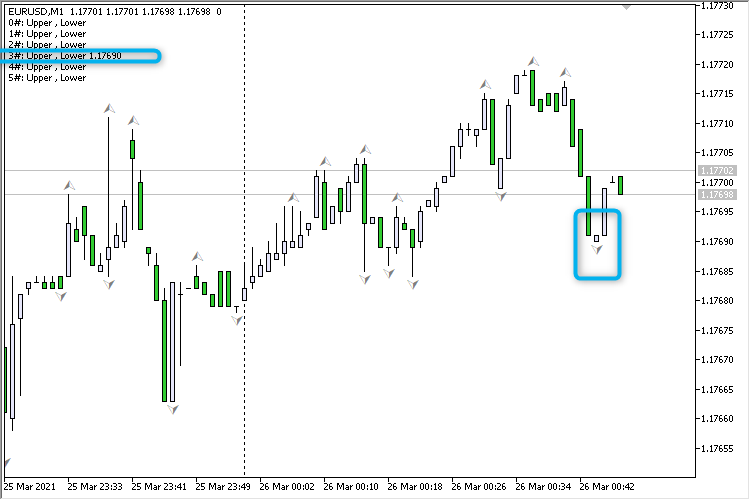# How to comment the values for Fractals?

This is what I have for using iFractals but I'm not able to comment the values of them properly as per the chart. I don't think I understand how to use those % symbols to get the values that I'm looking for. The following gives me some random values that doesn't reflect as per the indicator from the chart.

```double UpperFractalsArray[], LowerFractalsArray[];
int FractalsDefinition = iFractals(_Symbol,PERIOD_M1);
ArraySetAsSeries(UpperFractalsArray, true);
ArraySetAsSeries(LowerFractalsArray, true);
CopyBuffer(FractalsDefinition,UPPER_LINE,1,3,UpperFractalsArray);
CopyBuffer(FractalsDefinition,LOWER_LINE,1,3,LowerFractalsArray);

Comment(StringFormat("Test v4\nEntry : %s\nFirst 3 Upper Fractals : %3g %3g %3g\nFirst 3 Lower Fractals : %d %d %d",entry, UpperFractalsArray, UpperFractalsArray, UpperFractalsArray, LowerFractalsArray, LowerFractalsArray, LowerFractalsArray));```

`   int FractalsDefinition = iFractals(_Symbol,PERIOD_M1);`

Perhaps you should read the manual, especially the examples.
How To Ask Questions The Smart Way. (2004)
RTFM and STFW: How To Tell You've Seriously Screwed Up.

They all (including iFractals) return a handle (an int). You get that in OnInit. In OnTick/OnCalculate (after the indicator has updated its buffers), you use the handle, shift and count to get the data.
Timeseries and Indicators Access / CopyBuffer - Reference on algorithmic/automated trading language for MetaTrader 5
How to start with MQL5 - General - MQL5 programming forum - Page 3 #22 (2020)
How to start with MQL5 - MetaTrader 5 - General - MQL5 programming forum - Page 7 #61 (2020)
How to call indicators in MQL5 - MQL5 Articles (2010)

This is what I have for using iFractals but I'm not able to comment the values of them properly as per the chart. I don't think I understand how to use those % symbols to get the values that I'm looking for. The following gives me some random values that doesn't reflect as per the indicator from the chart.

Yes, Fractals can contain '0.0' or 'EMPTY_VALUE'. Example:

Code: 'iFractals.mq5'

```//+------------------------------------------------------------------+
//|                                                    iFractals.mq5 |
//+------------------------------------------------------------------+
#property version   "1.000"
//--- input parameters
input int         InputBars=9;
//---
int      handle_iFractals;             // variable for storing the handle of the iFractals indicator
bool     m_init_error      = false;    // error on InInit
//+------------------------------------------------------------------+
//| Expert initialization function                                   |
//+------------------------------------------------------------------+
int OnInit()
{
//--- forced initialization of variables
m_init_error            = false;    // error on InInit
//--- create handle of the indicator iFractals
handle_iFractals=iFractals(Symbol(),Period());
//--- if the handle is not created
if(handle_iFractals==INVALID_HANDLE)
{
//--- tell about the failure and output the error code
PrintFormat("Failed to create handle of the iFractals indicator for the symbol %s/%s, error code %d",
Symbol(),
EnumToString(Period()),
GetLastError());
//--- the indicator is stopped early
m_init_error=true;
return(INIT_SUCCEEDED);
}
//---
return(INIT_SUCCEEDED);
}
//+------------------------------------------------------------------+
//| Expert deinitialization function                                 |
//+------------------------------------------------------------------+
void OnDeinit(const int reason)
{
//---
}
//+------------------------------------------------------------------+
//| Expert tick function                                             |
//+------------------------------------------------------------------+
void OnTick()
{
if(m_init_error)
return;
//---
double upper[],lower[];
ArraySetAsSeries(upper,true);
ArraySetAsSeries(lower,true);
int start_pos=0,count=6;
if(!iGetArray(handle_iFractals,UPPER_LINE,start_pos,count,upper) || !iGetArray(handle_iFractals,LOWER_LINE,start_pos,count,lower))
return;
//---
string text="";
for(int i=0; i<count; i++)
{
//---
string text_upper="";
if(upper[i]==0.0 || upper[i]==EMPTY_VALUE)
text_upper="";
else
text_upper=DoubleToString(upper[i],Digits());
//---
string text_lower="";
if(lower[i]==0.0 || lower[i]==EMPTY_VALUE)
text_lower="";
else
text_lower=DoubleToString(lower[i],Digits());
//---
text=text+IntegerToString(i)+"#: "+"Upper "+text_upper+", Lower "+text_lower+"\n";
}
Comment(text);
}
//+------------------------------------------------------------------+
//| Get value of buffers                                             |
//+------------------------------------------------------------------+
bool iGetArray(const int handle,const int buffer,const int start_pos,
const int count,double &arr_buffer[])
{
bool result=true;
if(!ArrayIsDynamic(arr_buffer))
{
PrintFormat("ERROR! EA: %s, FUNCTION: %s, this a no dynamic array!",__FILE__,__FUNCTION__);
return(false);
}
ArrayFree(arr_buffer);
//--- reset error code
ResetLastError();
//--- fill a part of the iBands array with values from the indicator buffer
int copied=CopyBuffer(handle,buffer,start_pos,count,arr_buffer);
if(copied!=count)
{
//--- if the copying fails, tell the error code
PrintFormat("ERROR! EA: %s, FUNCTION: %s, amount to copy: %d, copied: %d, error code %d",
__FILE__,__FUNCTION__,count,copied,GetLastError());
//--- quit with zero result - it means that the indicator is considered as not calculated
return(false);
}
return(result);
}
//+------------------------------------------------------------------+
```

Result: